# Gravity: A Lua SDL Game

 [ LiB ]

### Gravity : A Lua SDL Game

I first introduced SDL way back in Chapter 4, where you used it with Python to do some pretty amazing stuff. Lua's SDL bindings aren't quite as complete, and unfortunately they are also a little out-of-date. The bindings are still in beta (Version 0.3 as of this writing) and were put together using the Lua 4 interpreter (the binary module has been pre-packaged with the toLua tool). Because of this, all of the necessary Lua scripts are bundled with the game inside the folder (so you don't try running it with Lua 5).

LuaSDL comes bundled with a 2D sprite game prototype called Meteor Shower . The game is written entirely in Lua and SDL by Thatcher Ulrich, who has generously given the source code to the public domain. I use this code as a base for Gravity . The entire source sample can be found in the Gravity folder in the Chapter 7 section on the CD, along with the pre-compiled DLLs necessary to use SDL and the Lua 4 interpreter.

You can launch Gravity from the command line; just navigate to the directory using the command line and type:

` Lua Gravity.lua `

In Gravity , the player is the moon in a universe gone haywire. Planetary objects and space travelers zoom across the screen, each attracted to themselves and to the player by their given mass (see Figure 7.1). The player must avoid these objects or face destruction.

##### Figure 7.1. Gravity goes haywire in this LuaSDL game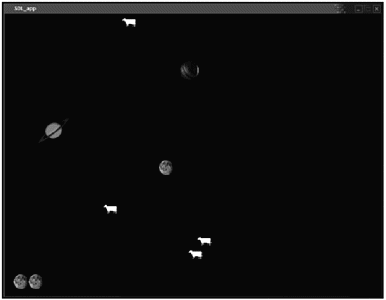A number of functions keep Gravity going. The list of functions for Gravity is shown in Figure 7.2.

##### Figure 7.2. The function list for Gravity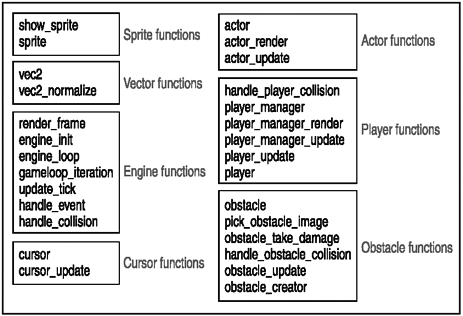#### Importing SDL

Before other code can start working, the program must have access to LuaSDL. This can be achieved with only a few short lines:

` -- Need to load the SDL module if loadmodule then         loadmodule("SDL") end `

NOTE

Lua 5 versus Lua 4

Lua 5.0 was released early in April of 2003. A number of new features came with Lua 5.0, including the following:

• Coroutines for executing many independent threads.

• Block comments for having multiple comment lines in code.

• Boolean types for true and false.

• Lightweight userdata that holds a value and not an object.

• Weak tables that assist with garbage collection.

• A faster virtual machine that is register-based.

• Standard libraries that use namespaces, although basic functions are still global.

• New methods of garbage collection, such as metamethods and other new features that make collection safe.

Along with the added features came a number of incompatibilities with previous Lua versions. Watch out for the following differences if you are a Lua 4.0 guru moving to Lua 5.0:

• Metatables have replaced the tag-method scheme.

• There are a number of changes to function calls.

• There are new reserved words (including false and true ).

• Most library functions are now defined inside Lua tables.

• lua_pushuserdata is deprecated and has been replaced with lau_newuserdata and lua_pushlightuserdata .

Work on 5.1 has already begun, and the rumor mill has it that this next version may be available by the end of 2003.

#### Setting Initial Variables

You must initialize a blit surface and a start gamestate early on for this 2D game.

Blitting , as you may recall from Chapter 4, is basically rendering or drawing, and in particular is the act of redrawing an object by copying the pixels of an object onto the screen.

An SDL blit surface looks like this:

` SDL.SDL_BlitSurface = SDL.SDL_UpperBlit; `

The gamestate is a collection of state variables, assigned to a Lua table, that are initialized before the game starts to run. These are listed in Table 7.1.

##### Table 7.1. The gamestate Variables

Element

Value

last_update_ticks

begin_time

elapsed_ticks

frames

update_period

30

active

1

new_actors

Nested table

actors

Nested table

Function

` gamestate = {         last_update_ticks = 0,         begin_time = 0,         elapsed_ticks = 0,         frames = 0,         update_period = 30,     -- interval between calls to update_tick         active = 1,         new_actors = {},         actors = {},         add_actor = function(self, a)                 assert(a)                 tinsert(self.new_actors, a)         end } `

In this table there are a number of variables set to 0 and also a few nested tables. The update_period is the interval in milliseconds between calls to the update tick, and active is a Boolean that says whether the engine is currently active or not. The add_actor function is also defined in this table.

The next Lua table is for a sprite cache. This cache will hold sprites that have already been loaded, so the engine won't have to try and load them on-the-fly :

` sprite_cache = {} `

Gravity is all about speed and velocity and, well, gravity. I envisioned flying planetary objects, each with different masses, bumping and colliding with each other in a solar system-like playing screen. To achieve this effect, I have to set gravity, how often obstacles fly onto the screen, and how many lives the player will have.

` -- Set gravity GRAVITY_CONSTANT = 100000 -- table of virtual masses for the different obstacle sizes obstacle_masses = { 10, 50, 75 } OBSTACLE_RESTITUTION = .05 -- soft speed-limit on obstacles SPEED_TURNOVER_THRESHOLD = 4000 -- player manager actor MOONS_PER_GAME = 3 --How often till new obstacle appears BASE_RELEASE_PERIOD = 500 `

The three obstacles, two planets and a space cow, are illustrated in Figure 7.3. Each will use a unique bitmap image that is already included in the Gravity folder. These images are placed into a Lua table.

##### Figure 7.3. The three obstacles in Gravity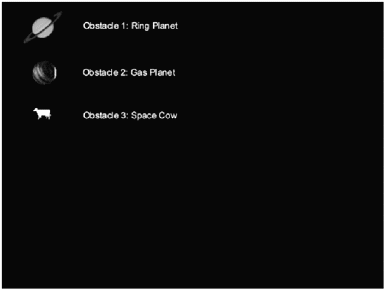` --load the bitmap obstacle images obstacle_images = {         { "obstacle1.bmp" },         { "obstacle2.bmp" },         { "obstacle3.bmp" }, } `

#### Creating Functions

Creating functions is really the meat and gravy of the endeavor. You need functions, lots of functions. Sprites, vectors, events, the game engine, and each actor (or object) within the game must be handled.

##### Sprite Handling

Sprite handling is the first thing to tackle (see Figure 7.4). The main sprite function will be a constructor that takes in a bitmap file and returns an SDL surface that can be blitted and used by the engine. A function that draws the new blitted SDL surface sprite onto a rect ( rects are again from Chapter 4they are the basic object for a 2D SDL game) will be part of the process as well. The main sprite function will be sprite() :

##### Figure 7.4. Sprite handling functions in Gravity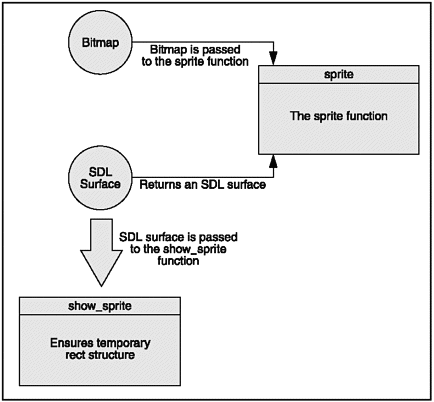` function sprite(file) -- The sprite constructor. Passes in a bitmap filename and returns an SDL_Surface         --First check the cache         if sprite_cache[file] then                 return sprite_cache[file]         end          local temp, my_sprite;         -- Load the sprite image         my_sprite = SDL.SDL_LoadBMP(file);         if my_sprite == nil then                 print("Couldn't load " ..  file .. ": " .. SDL.SDL_GetError());                 return nil         end         -- Set colorkey to black (for transparency)         SDL.SDL_SetColorKey(my_sprite, SDL.bit_or(SDL.SDL_SRCCOLORKEY, SDL.SDL_RLEACCEL), 0)         -- Convert sprite to video SDL format         temp = SDL.SDL_DisplayFormat(my_sprite);         SDL.SDL_FreeSurface(my_sprite); my_sprite = temp;         sprite_cache[file] = my_sprite         return my_sprite end `

The sprite constructor first checks to make sure that the sprite doesn't already exist in sprite_cache . If it does not, the constructor tries to find the given BMP image file. If the file doesn't exist, the constructor exits with an error; otherwise it goes ahead and loads the image into an SDL format (using a temp variable as interim), sets the colorkey (another Chapter 4 concept), loads the sprite into the sprite_cache , and returns the sprite.

The second sprite function, show_sprite , is passed a sprite and draws it on the screen at the given coordinates (x,y). It uses the massively powerful rect() to accomplish this. Notice that in order for show_sprite to work, it needs all four variables:

` function show_sprite(screen, sprite, x, y)         -- make sure we have a temporary rect structure         if not temp_rect then                 temp_rect = SDL.SDL_Rect_new()         end         temp_rect.x = x - sprite.w / 2         temp_rect.y = y - sprite.h / 2         temp_rect.w = sprite.w         temp_rect.h = sprite.h         SDL.SDL_BlitSurface(sprite, NULL, screen, temp_rect) end `

##### Vector Handling

When used in game physics, vectors combine magnitude (speed) and direction (see Figure 7.5). Vectors are extremely useful, as the engine needs to know the speed and direction of the objects and actors flying around the screen. In order to do this, the vec2 function needs to take in a table and do some math.

##### Figure 7.5. Vectors in physics combine magnitude and direction.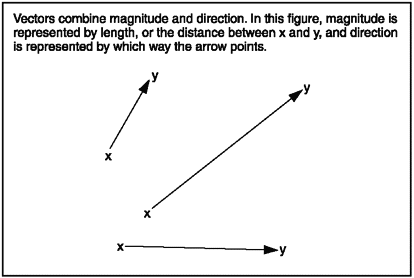In geometry, vectors consist of a point or a location in space, a direction, and distance. The combination of direction and distance is sometimes called displacement . The vec2 function helps to keep track of vectors using x and y coordinates, as shown in Figure 7.6. The starting coordinates are a.x and a.y , and the ending coordinates are b.x and b.y .

##### Figure 7.6. Starting and ending points of a vectorThe vec2 function has a number of methods for determining speed and direction of an actor or object using vectors. The add , sub , mul , and unm methods are used to track position in two-dimensional space by performing sector arithmetic.

The add method is used to do vector addition where the results of two vectors can be plotted in two-dimensional space, as shown in Figure 7.7. Vector subtraction is handled by the sub method, and does the opposite of vector addition by delivering the difference between two vectors.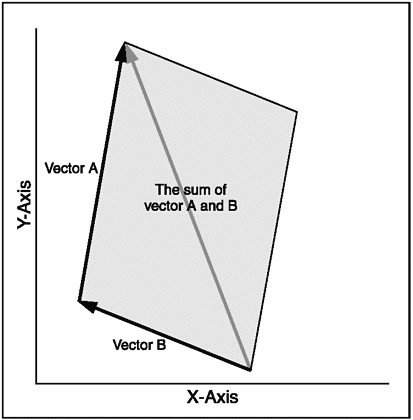You can multiply a vector by a constant to produce a second vector that travels in the same or the opposite direction but at a different speed. Multiplying vectors in math is called scalar multiplication . Scalar multipication can be really useful for collisionssay if two planets in the Gravity game collide, and they need to bounce off of each other in opposite directions.

There is also a second way of multiplying vectors that gives the angle between two vectors. This called the dot product ; it is also handled by the mul method. Although you don't use the dot product in this game, it is a useful vector function and is sometimes used to perform lighting calculations (say, if you wanted to add a sun object that casts shadows to the game) or determine facing in 3D games .

After running through vec2 , vec2_normalize finishes the vector math by dividing by the length and catching any possible close to 0 calculations that could cause errors.

` --vec2_tag = nil -- re-initialize the vector type when reloading function vec2(t) -- constructor         if not vec2_tag then                 vec2_tag = newtag()                 Vector addition                 settagmethod(vec2_tag, "add",                         function (a, b) return vec2{ a.x + b.x, a.y + b.y } end                 )                 Vector subtraction                 settagmethod(vec2_tag, "sub",                         function (a, b) return vec2{ a.x - b.x, a.y - b.y } end                 )                 Vector multiplication                 settagmethod(vec2_tag, "mul",                         function (a, b)                                 if tonumber(a) then                                         return vec2{ a * b.x, a * b.y }                                  elseif tonumber(b) then                                         return vec2{ a.x * b, a.y * b }                                 else                                         -- dot product.                                         return (a.x * b.x) + (a.y * b.y)                                 end                         end                 )                 settagmethod(vec2_tag, "unm",                         function (a) return vec2{ -a.x, -a.y } end                 )         end         local v = {}         if type(t) == 'table' or tag(t) == vec2_tag then                 v.x = tonumber(t) or tonumber(t.x) or 0                 v.y = tonumber(t) or tonumber(t.y) or 0         else                 v.x = 0                 v.y = 0         end         settag(v, vec2_tag)         v.normalize = vec2_normalize         return v end function vec2_normalize(a) -- If a has 0 or near-zero length, sets a to an arbitrary unit vector         local d2 = a * a         if d2 < 0.000001 then                 -- Return arbitrary unit vector                 a.x = 1                 a.y = 0         else                 -- divide by the length to get a unit vector                 local length = sqrt(d2)                 a.x = a.x / length                 a.y = a.y / length         end end `

##### Event Handling

Handlers for key presses and mouse clicks are necessary for any computer game. Mouse events will be picked up by the individual actor that controls the player, but monitoring for the keyboard and windows events must also occur in case a player wants to close a window or quit using the Escape key. This can be done fairly easily (see Figure 7.8) by using SDL_KEYDOWN to watch for SDLK_q or SDLK_ESCAPE .

##### Figure 7.8. Event handling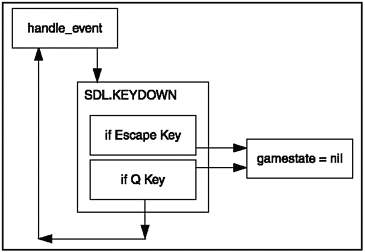` function handle_event(event) -- called by main loop --Checks for keypresses -- sets gamestate to nil if player wants to quit         if event.type == SDL.SDL_KEYDOWN then                 local   sym = event.key.keysym.sym                 if sym == SDL.SDLK_q or sym == SDL.SDLK_ESCAPE then                         gamestate.active = nil                 end         elseif event.type == SDL.SDL_QUIT then                 gamestate.active = nil         end end `

##### The Engine and the Game Loop

A number of actions must happen in the engine and game loop, and these actions should correspond to a codeable function. You must have a function to remove any sprites that aren't being used and add any new ones, a function to render the screen and background, a function that keeps track of time and updates the game state, a function that does the blitting, and a function that listens for player keystrokes:

• engine_init. Sets screen and video.

• engine_loop. Main engine loop.

• gameloop_iteration. Tracks time and call other functions.

• update_tick. Updates any game actors.

• handle_event. Listens for any events caused by the player.

• handle_collision. Handles any actor collisions.

The first step is to initialize the engine.

The engine_init function is used to set the screen width and height and the video mode and to start the game ticking, so to speak. It does all this through common-sense local variables, a few SDL calls, and calling gamestate :

` function engine_init(argv)         local width, height;         local video_bpp;         local videoflags;         videoflags = SDL.bit_or(SDL.SDL_HWSURFACE, SDL.SDL_ANYFORMAT)         width = 800         height = 600         video_bpp = 16         -- Set video mode         gamestate.screen = SDL.SDL_SetVideoMode(width, height, video_bpp, videoflags);         gamestate.background = SDL.SDL_MapRGB(gamestate.screen.format, 0, 0, 0);         SDL.SDL_ShowCursor(0)         -- initialize the timer/ticks         gamestate.begin_time = SDL.SDL_GetTicks();         gamestate.last_update_ticks = gamestate.begin_time; end `

Removing any actors that are no longer used and adding any new actors is handled by an update_tick function. Two Lua for loops iterate through each actor in the game. The first removes any actors that aren't active and adds any new ones:

` for i = 1, getn(gamestate.actors) do         if gamestate.actors[i].active then                         -- add the actors                         tinsert(gamestate.new_actors, gamestate.actors[i])         end end `

The former gamestate.actor table is then replaced with the new table in a quick swap:

` gamestate.actors = gamestate.new_actors gamestate.new_actors = {} `

Then a second for loop calls an update for each actor in the table:

` -- call update for each actor         for i = 1, getn(gamestate.actors) do                 gamestate.actors[i]:update(gamestate)         end `

After the actors have been updated, each needs to be redrawn, as does the screen. A quick render_frame function does this work, first clearing the current screen and then redrawing each actor rect() within gamestate.actors :

` function render_frame(screen, background) -- When called renders a new frame.         -- First clears the screen         SDL.SDL_FillRect(screen, NULL, background);         -- re-draws each actor in gamestate.actors         for i = 1, getn(gamestate.actors) do                 gamestate.actors[i]:render(screen)         end         -- updates         SDL.SDL_UpdateRect(screen, 0, 0, 0, 0) end `

Most of the actual game-engine work is done by this next little function, called gameloop_iteration . It is called each time the engine loops, and is responsible for calling all the other rendering functions and keeping track of time. First gameloop_iteration calls handle_event on any pending events in the gamestate's event_buffer (checking first that the buffer exists):

` function gameloop_iteration() -- call this to update the game state.  Runs update ticks and renders -- according to elapsed time.         -- if buffer doesnt exist make it so         if gamestate.event_buffer == nil then                 gamestate.event_buffer = SDL.SDL_Event_new()         end         -- run handle_even on any pending events         while SDL.SDL_PollEvent(gamestate.event_buffer) ~= 0 do                 handle_event(gamestate.event_buffer)         end `

gameloop_iteration then uses SDL_GETTICKS() to set the local time variable and compares this with the gamestate to see if an update needs to occur. If the engine needs to update, then update_tick is called and the time count is updated:

` -- run any necessary updates         local time = SDL.SDL_GetTicks();         local delta_ticks = time - gamestate.last_update_ticks         local update_count = 0         while delta_ticks > gamestate.update_period do                 update_tick();                 delta_ticks = delta_ticks - gamestate.update_period                 gamestate.last_update_ticks = gamestate.last_update_ticks +  gamestate.update_period                 update_count = update_count + 1         end `

Finally, render_frame has to be called to redraw any actors and the screen background if an update has occurred:

` -- if we did any updates, then render a frame         if update_count > 0 then                 render_frame(gamestate.screen, gamestate.background)                 gamestate.frames = gamestate.frames + 1         end end `

The actual engine game loop ( engine_loop ) runs while the gamestate is active. The engine_loop calls gameloop_iteration each time its own while loop fires. The engine_loop then cleans out the buffer. If the gamestate is no longer active, then engine_loop calls SDL_QUIT :

` function engine_loop() -- While loop calls gameloop_iteration         while gamestate.active do                 gameloop_iteration()         end         -- clean up         if event_buffer then                 SDL.SDL_Event_delete(event)         end         SDL.SDL_Quit(); end `

##### Actors

Everyone wants to be an actoror a computer game programmerthese days. Actors in Gravity aren't as revered or lucky as the Hollywood variety, however. They are the constructs that can be interacted with in the game, as shown in brief in Figure 7.9. These base actor functions will be used by the other objects in the game.

##### Figure 7.9. Actors are initialized in GravityLearning how to update an actor's position on the screen is the first task here, and this is where the vector functions get to stretch their legs. Velocity is multiplied by how much time has elapsed in the gamestate loop since the last update:

` function actor_update(self, gs) -- Updates than actor using vector functions         local   dt = gamestate.update_period / 1000.0         -- update according to velocity & time         local   delta = self.velocity * dt         self.position = self.position + delta `

Since this is a 2D Asteroids -type game, objects on the screen should wrap around to the other side when they hit an edge. This effect is achieved with simple math applied to the position and the game screen ( gs.screen ) before actor_update ends:

` -- wrap around at screen edge         if self.position.x < -self.radius and self.velocity.x <= 0 then                 self.position.x = self.position.x + (gs.screen.w + self.radius * 2)         end         if self.position.x > gs.screen.w + self.radius and self.velocity.x >= 0 then                 self.position.x = self.position.x - (gs.screen.w + self.radius * 2)         end         if self.position.y < -self.radius and self.velocity.y <= 0 then                 self.position.y = self.position.y + (gs.screen.h + self.radius * 2)         end         if self.position.y > gs.screen.h + self.radius and self.velocity.y >= 0 then                 self.position.y = self.position.y - (gs.screen.h + self.radius * 2)         end end `

A function that blits actors onto the screen using show_sprite is the next thing to create after determining the actor's position:

` function actor_render(self, screen) -- Blit the given actor to the given screen         show_sprite(screen, self.sprite, self.position.x, self.position.y) end `

The final curtain on actors is to build an actor constructor. The constructor will take in the sprite bitmap and keep track of position, velocity, and radius, and then return the actor in a nice, neat Lua table:

` function actor(t) -- actor constructor.  Pass in the name of a sprite bitmap.         local a = {}         -- copy elements of t         for k,v in t do                 a[k] = v         end         a.type = "actor"         a.active = 1         a.sprite = (t or t.sprite and sprite(t or t.sprite)) or nil         a.position = vec2(t.position)         a.velocity = vec2(t.velocity)         a.radius = a.radius                 or (a.sprite and a.sprite.w * 0.5)                 or 0         a.update = actor_update         a.render = actor_render         return a end `

##### Obstacles

The game obstacles are cows and planets. These obstacles must track a number of different things in order to make the game interesting.

• Obstacles can take damage. Some of the bigger objects will survive collisions with several smaller objects, so they need to track how much damage they can take.

• Obstacles need to know when they collide with something.

• Obstacles are drawn to each other by gravity, and so they need to keep track of other nearby obstacles.

Obstacles should also occasionally appear on the screen. They should come from offscreen at a random place, at a random speed, and travel somewhat towards the center of the screen. These object capabilities are handled with the following functions:

• obstacle_update(). Handles gravity, movement, and collisions.

• handle_obstacle_collision(). Called when a collision is detected .

• obstacle_take_damage(). Damages the object.

• pick_obstacle_image(). Chooses one of the obstacle images at random.

• obstacle(). The obstacle constructor.

• obstacle_creator(). Randomly places obstacles onto the screen.

The obstacle_update is the first function to tackle. It watches for collisions by first updating itself and then keeping track of where the other actors are:

` function obstacle_update(self, gs) -- update this obstacle.  watch for collisions with other actors.         -- move ourself         actor_update(self, gs)         local   dt = gamestate.update_period / 1000         local   accel = vec2()         -- check for the position of other actors         for i = 1, getn(gs.actors) do                 local   a = gs.actors[i] `

Actors with a large mass will draw other actors towards themselves. This is simulated with the GRAVITY_CONSTANT , the two actors' mass, and some math.

The Newtonian concept of attraction takes the mass of two objects, the distance between them, and the constant of gravity to determine how strong the attraction is between the two objects (see Figure 7.10).

##### Figure 7.10. Newton's law of attraction (i.e. universal gravitation )This law is usually expressed by (G*m1)*(G*m2)/r^2, where G is the gravitational constant, m1 is the mass of the first object, m2 is the mass of the second object, and r is the distance between the two objects.

This formula is used in obstacle_update by taking the GRAVITY_CONSTANT and the mass of an object ( a.mass ) and accelerating actors towards other actors:

` -- if the actor has mass then compute a gravitational acceleration towards it         if a.mass then                 local r = a.position - self.position                 local d2 = r * r                  if d2 < 100 * 100 then                         local d = sqrt(d2)                         if d * 2 > self.radius then                             accel = accel + r * ((GRAVITY_CONSTANT * a.mass) / (d2 * d))                         end                 end         end `

Then obstacle_update needs to check for actual collisions and handle them by calling handle_collision . You end the function by resetting the actor's velocity:

` -- check for collisions, and respond                 if a and a ~= self and a.collidable then                         local disp = a.position - self.position                         local distance_squared = disp * disp                         local sum_radius_squared = (a.radius + self.radius) ^ 2                         if distance_squared < sum_radius_squared then                                 -- we have a collision, call the collision handler.                                 handle_collision(self, a)                         end                 end         end         self.velocity = self.velocity + accel * dt end `

The next function, handle_obstacle_collision , fires when the obstacles collide. It first makes sure that the collision is between two obstacles and not between an obstacle and the player; that would be handled by a different function. It then damages the objects that collide by calling obstacle_take_damage :

` function handle_obstacle_collision(a, b) -- handles a collision between two obstacles, a and b.         --Make sure we are handling collison between two obstacles, otherwise exit         if a.type == "obstacle" and b.type == "obstacle" then            -- impulse will be along the displacement vector between the two obstacles            local normal = b.position - a.position            normal:normalize()            local relative_vel = b.velocity - a.velocity            -- Damage the objects that collide            local collisionenergy = 0.1 * (relative_vel * realtive_ve;) * (a.mass + b.mass)            local split_dir = vec2{ normal.y, -normal.x }            obstacle_take_damage(a, split_dir, -normal, collision_energy)            obstacle_take_damage(b, split_dir, normal, collision_energy)         end end `

The obstacle_take_damage is called in the event of a collision. Some objects may survive a collision, but at least one (the one with lesser mass) will be destroyed. The smallest objects (cows) will always be destroyed :

` function obstacle_take_damage(a, split_direction, collision_normal, collision_energy) -- damage the obstacle; if it's damaged enough, destroy         local split_speed = sqrt(2 * collision_energy / a.mass) * 0.35         -- obstacle takes damage; when its damage reaches 0 it dies         a.hitpoints = a.hitpoints - collision_energy / 2000         if a.hitpoints > 0 then                 -- collision is not violent enough to destroy this obstacle                 return         end         local new_size = a.size - 1         if new_size < 1 then                 -- The smallest obstacle always disintegrates.                 a.active = nil                 return         end         -- kill a         a.active = nil end `

Pick_obstacle_image is a short random function that will pick which object to use from the image_table using Lua's built-in random :

` function pick_obstacle_image(size)         local image_table = obstacle_images[size]         -- pick one of the obstacle images at random         return image_table[random(getn(image_table))] end `

The obstacle constructor uses the actor constructor as its building block. It then sets its type to "obstacle" , flags it as collideable, makes sure it has one of the three obstacle sizes, and then sets variables for radius, size, and speed. It also assigns the obstacle to obstacle_update :

` -- constructor -- start with a regular actor       local a = actor(t)       a.type = "obstacle"       a.collidable = 1       a.size = a.size or 3      -- make sure caller defined one of the three sizes of obstacle       a.sprite = sprite(pick_obstacle_image(a.size))       a.radius = 0.5 * a.sprite.w       a.mass = obstacle_masses[a.size]       a.hitpoints = a.mass * a.mass          -- implement a speed-limit on obstacles         local   speed = sqrt(a.velocity * a.velocity)         if speed > SPEED_TURNOVER_THRESHOLD then                 local new_speed = SPEED_TURNOVER_THRESHOLD + sqrt(speed - SPEED_TURNOVER_THRESHOLD)                 a.velocity = a.velocity * (new_speed / speed)         end         -- attach the behavior handlers         a.update = obstacle_update         return a end `

Math functions like sqrt() have a reputation for being slow, especially when complex math has to be calculated on-the-fly. Having to process sudden large computations can cause an otherwise fluidly running game to grind to a halt. One way to speed up sqrt is to cache any square root values that are used more than once. Let's say you had the following code:

` a* sqrt(s) b* sqrt(s) c =  a+b `

Instead of running the sqrt() function twice, run it once first and store the value:

` square = sqrt(s) a*square b*square c = a+b `

A second trick is to do common math ahead of time and place it in a table for the program. Let's say you did a log of power of multiplication in a program; you could work out common equations first and put them in a table like Table 7.2.

##### Table 7.2. Common Power

Initial Value

^2

^ 3

2

4

8

3

9

27

4

16

64

5

25

125

6

36

216

When the code needs one of these values, it gets a reference to the appropriate row and column instead of calculating on-the-fly.

The very last thing obstacles need to do is appear occasionally on the screen to harass the player. This is achieved by creating an actor that sets a countdown timer. When the timer reaches 0, the actor calls the obstacle construct, creates the obstacle on the edge of the screen, and sets it flying towards the middle somewhere. Then it starts the timer over again:

` -- random obstacle creator function obstacle_creator(t) -- constructs an actor that randomly spawns a new obstacle periodically         a = {}         a.active = 1         a.type = "obstacle_creator"         a.collidable = nil         a.position = vec2{ 0, 0 }         a.velocity = vec2{ 0, 0 }         a.sprite = nil         -- set the random timer countdown         a.period = t.period or t or 100      -- period between spawning obstacles         a.countdown = a.period         a.render = function () end         a.update =                 function (self, gs)                         self.countdown = self.countdown - gs.update_period                         if self.countdown < 0 then                                 -- timer has expired; spawn an obstacle                                 -- pick a random spot around the edge of the screen                                 local w, h = gs.screen.w, gs.screen.h                                 local edge = random(w * 2 + h * 2)                                 local pos                                 if edge < w then                                         pos = vec2{ edge, 0 }                                 elseif edge < w*2 then                                         pos = vec2{ edge - w, h }                                 elseif edge < w*2 + h then                                         pos = vec2{ 0, edge - w*2 }                                 else                                         pos = vec2{ w, edge - (w*2 + h) }                                 end                                 -- aim at the middle of the screen                                 local vel = vec2{ w/2, h/2 } - pos                                 vel:normalize()                                 vel = vel * (random(400) + 50)                                 gs:add_actor(                                         obstacle{                                                 size = random(3),                                                 position = pos,                                                 velocity = vel                                         }                                 )                                 -- reset the timer                                 self.countdown = self.period                         end                 end         return a end `

##### The Player

The player is arguably the most important game piece. Much of the infrastructure the player needs (such as sprite handling and actor functions) has already been laid out. However, you still need functions to handle the following:

• Updating the player

• Player collision

• The player constructor

The player_updater function handles updating the player; it looks similar to the object_updater function. The player object is handled just like an operating system's mouse cursor. The player's position is based on the mouse position. Using SDL_GetMouseState , the player position is updated, and checks for any collisions are made. If there is a collision, handle_player_collision is called:

` function player_update(self, gs) -- update the player and watch for collisions         local   dt = gamestate.update_period / 1000         -- get the mouse position, and move the player position towards the mouse position         local   m = {}         m.buttons, m.x, m.y = SDL.SDL_GetMouseState(0, 0)         local   mpos = vec2{ m.x, m.y }         local   delta = mpos - self.position         local   accel =                 delta * 50      -- move towards the mouse cursor                 - self.velocity * 10    -- damping         self.velocity = self.velocity + accel * dt         -- move ourself         actor_update(self, gs)         -- check for collisions against all other actors         for i = 1, getn(gs.actors) do                 local   a = gs.actors[i]                 -- check for collisions, and respond                 if a and a ~= self and a.collidable then                         local disp = a.position - self.position                          local distance_squared = disp * disp                         local sum_radius_squared = (a.radius + self.radius) ^ 2                         if distance_squared < sum_radius_squared then                                 -- we have a collision                                 -- call the collision handler.                                 handle_player_collision(self, a)                         end                 end         end end `

The handle_player_collision also looks quite a bit like the handle_obstacle_collision, except it's shorter because there is no concern over damage. A collision will kill the player by setting its active method to nil :

` function handle_player_collision(a, b) -- handles a collision between a player, a, and some other object, b         -- impulse will be along the displacement vector between the two obstacle         local normal = b.position - a.position         normal:normalize()         local relative_vel = b.velocity - a.velocity         if relative_vel * normal >= 0 then                 -- don't do collision response if obstacles are moving away from each other                 return         end         -- Kill the player         a.active = nil end `

The player constructor is similar to the other constructors that have been built, except that it's smaller. The actor template is used initially, then the constructor loads the moon.bmp as its image, sets itself as collideable, gives itself a mass (yes, the player's gravity attracts objects) and radius, and sets itself to run player_update .

` function player(t) -- constructor         -- start with a regular actor         local a = actor(t)         a.type = "player"         a.collidable = 1         a.sprite = sprite("moon.bmp") -- or error("can't load ....")         a.radius = 0.5 * a.sprite.w         a.mass = 10         -- attach the behavior handlers         a.update = player_update         return a end `

The player object needs a few utility functions with which to keep track of his lives and whether he's entered the game. The player cursor will have different visual states before the game starts, while playing, and after a collision, so these need to be kept track of as well. This is done with corresponding functions in the player_manager .

First is the player_manager_update . It keeps track of the player state, which is either pre-game or setup, active or playing, or deceased. If the player has died, player_manager_update checks to see if there are any lives left by checking the MOONS_PER_GAME constant. If there are, there is a short delay before the player can launch his next moon. These are all handled by a handful of Lua if elseif then statements:

` function player_manager_update(self, gs) -- keep track of game functions         if self.state == "pre-setup" then                 -- delay, and then enter setup mode.                 self.countdown = self.countdown - gamestate.update_period                 if self.countdown <= 0 then                         self.state = "setup"                         self.cursor.active = 1                         gamestate:add_actor(self.cursor)                 end         elseif self.state == "setup" then                 if not self.cursor.active then                         -- player has placed the moon.  start playing.                         self.player.active = 1                         self.player.position = self.cursor.position                         gamestate:add_actor(self.player)                         -- deduct the moon that we just placed.                         self.moons = self.moons - 1                         self.state = "playing"                 end         elseif self.state == "playing" then                 if not self.player.active then                         -- player has died.                         if self.moons <= 0 then                                 -- game is over                                 self.state = "pre-attract"                                 self.countdown = 1000                         else                                 -- set up for next moon                                 self.state = "pre-setup"                                 self.countdown = 1000                         end                 end         elseif self.state == "pre-attract" then                 -- delay, and then enter attract mode                 self.countdown = self.countdown - gamestate.update_period                  if self.countdown <= 0 then                         self.state = "attract"                 end         elseif self.state == "attract" then                 local m = {}                 m.buttons, m.x, m.y = SDL.SDL_GetMouseState(0, 0)                 if m.buttons > 0 then                         -- start a new game.                         self.state = "pre-setup"                         self.moons = MOONS_PER_GAME                         self.countdown = 1000                 end         end end `

The function called player_manager_render comes in at this point to display moon sprites that show how many lives the player has left:

` function player_manager_render(self, screen)         if self.state == "attract" then                 show_sprite(screen, self.game_over_sprite, screen.w / 2, screen.h / 2)         else                 -- show the moons remaining                 local sprite = self.player.sprite                 local x = sprite.w                 local y = screen.h - sprite.h                 for i = 1, self.moons do                         show_sprite(screen, sprite, x, y)                         x = x + sprite.w                 end         end end `

The player_manager constructor is the last function you need to wrap up the player. Like the constructors, this function builds a Lua table that stores the variable you need, such as which player mouse curser you currently use, how many lives are left, and who to call for rendering and updating:

` function player_manager(t) -- constructor         local a = {}         for k, v in t do a[k] = v end  -- copy values from t         a.active = 1         a.moons = MOONS_PER_GAME         a.state = "setup"         a.cursor = cursor{         }          gamestate:add_actor(a.cursor)         a.player = player{                 position = { gamestate.screen.w / 2, gamestate.screen.h / 2 },                 velocity = { 0, 0 },         }         a.obstacle_creator.period = BASE_RELEASE_PERIOD         a.game_over_sprite = sprite("finish.bmp")         a.update = player_manager_update         a.render = player_manager_render         return a end `

##### Starting the Game

Almost finished! Only a few functions remain . The mouse cursor must be properly tracked and you need a check for mouse buttons that will start gameplay. The mouse cursor is set initially to a start.bmp graphic that lets the player choose where to position the moon when in the playing window. All of these actions are accomplished with cursor_update and the cursor constructor, and all the information is held within Lua tables:

` function cursor_update(self, gs) -- update the cursor.  follow the mouse.         local   m = {}         m.buttons, m.x, m.y = SDL.SDL_GetMouseState(0, 0)         self.position.x = m.x         self.position.y = m.y         if m.buttons ~= 0 then                 -- player has clicked                 self.active = nil         end end function cursor(t) -- constructor         -- start with a regular actor         local a = actor(t)         a.type = "cursor"         a.sprite = sprite("start.bmp") -- or error("can't load ....")         a.radius = 0.5 * a.sprite.w         -- attach the behavior handlers         a.update = cursor_update         return a end `

Initializing the game engine is a pretty straightforward endeavor after all the work that's already been done. The engine_init function is called, and a slew of obstacles are in the gamestate with add_actor :

` engine_init{} -- Generate a bunch of obstacles for i = 1,10 do         gamestate:add_actor(                 obstacle{                         position = { random(gamestate.screen.w), random(gamestate.screen.h) },                         velocity = { (random()*2 - 1) * 100, (random()*2 - 1) * 100 }, - - pixels/sec                         size = random(3)                 }         ) end `

Then create an obstacle_creator and a player_manager and let them duke it out:

` -- create an obstracle creator creator = obstacle_creator{} gamestate:add_actor(creator) -- create a player manager gamestate:add_actor(         player_manager{                 obstacle_creator = creator         } ) `

Last but not least, call the engine_loop() , and lo-and-behold, the game is running:

` -- run the game engine_loop() `

 [ LiB ]Game Programming with Pyton, Lua and Ruby
ISBN: N/A
EAN: N/A
Year: 2005
Pages: 133